Disclosure: This post may contain affiliate links, meaning when you click the links and make a purchase, we receive a commission.

# Relative cell references in Excel

Before understanding relative cell references, you have to understand cell reference in a formula. Say I have a formula like this ‘=B3*C3+D3’. In this formula there are three references, they are B3, C3, and D3. Every reference in a formula links with a cell in excel worksheet. In another word, every cell has a unique reference. B3 can link to only one cell in the worksheet.

There are two types of references in excel-relative references and absolute references. Relative and absolute references behave differently when they are copied and filled with other cells. If you use relative references in a formula, the formula will change if you copy the formula to another cell. On the other hand, absolute references will remain constant, no matter in which they are copied.

## What is relative cell references

By default, all cell references are relative references. When you copy a formula with relative references in other cells, the references will change based on the relative positions of rows and columns. Say for example you have a formula like this: B4*C4+D4 in cell E4. Then E4=B4*C4+D4. If you copy this formula in cell E5 dragging AutoFill, then B4 will be B5, C4 will be C5 and D4 will be D5. One row down. What will be the references if you copy this formula(E4=B4*C4+D4) to cell F4? The formula will be now F4=C4*D4+E4. One column right.

Relative references are convenient when you need to repeat the same calculation across multiple columns or rows.

## Creating and Copying formula using relative references in Excel

In our following example, we want to calculate Menu Cost(see the heading in the example) multiplying every menu’s price and quantity. We shall not create the formula for every cell, we shall create the formula for cell D4 and copy this formula to the other rows. Here we are using relative references.

### Step 1:

Select the cell that will contain your formula. In our example, the cell is D4 where we shall use the formula.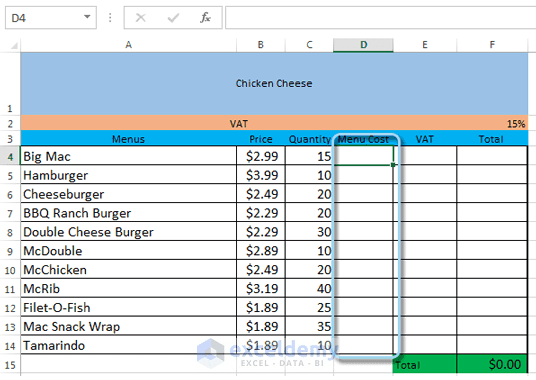Relative cell references in Excel. We shall calculate the Menu Cost using relative cell references

### Step 2:

Enter your formula to calculate the desired value in the selected cell. We shall type ‘=B4*C4’ in cell D4.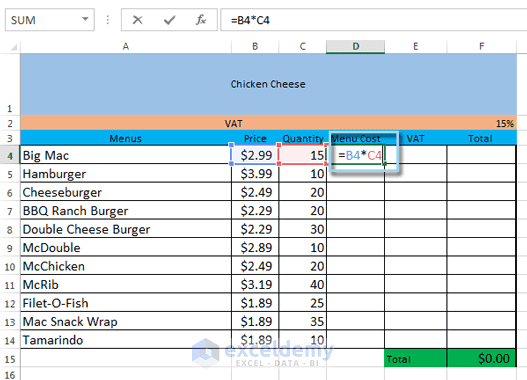Relative cell references in Excel. Entering Formula

### Step 3:

Now press Enter on your keyboard. The formula will be calculated internally and you will see the calculated result in cell D4.

### Step 4:

By default when you pressed Enter, the active cell is now D5. Use up arrow(↑) key to make D4 as active cell again. Now locate the fill handle in the lower-right corner of the cell D4.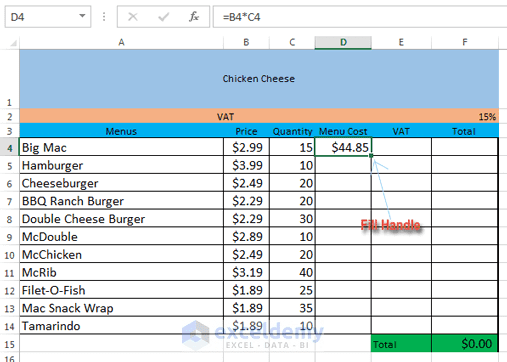Relative cell references in Excel. Fill Handle

### Step 5:

Now click the fill handle, hold it and drag over the cells you wish to fill. We are selecting cells D5: FormulaD14 in our example.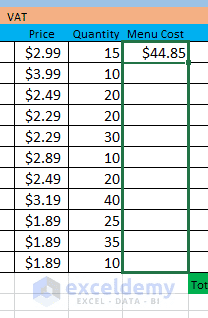Relative cell references in Excel. Fill Handle grabbing.

### Step 6:

Now release the mouse. The formula, we made in cell D4, will be copied to every cell we have selected with relative references. Calculated values will be visible in every cell.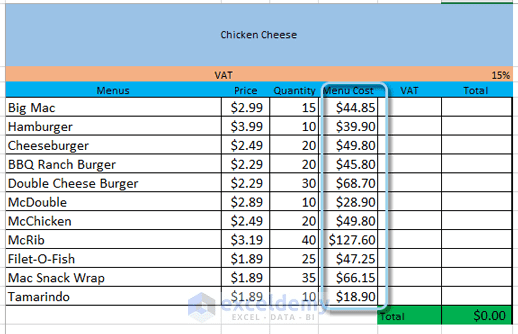Relative cell references in Excel. The formula is copied with relative cell references, values are visible now.

Note: To check whether your formula is correct, just double-click on any cell from the filled cells. You will find that this formula is similar to ‘=B4*C4’ formula, except that cell references are different and dependent on row numbers. For the row number 9, the formula is ‘=B9*C9’, just the row numbers have been changed.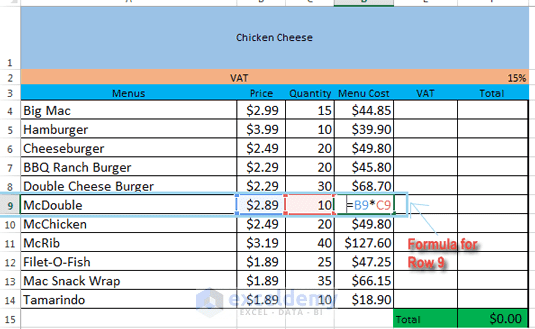Relative cell references in Excel. The formula is same, just the cell references have been changed with the row numbers.

relative-absolute-references.xlsx